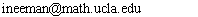2014 summer bootcamp in analysis.

Time and place: MTWTh 10:00am to 11:50am, MS 5138.

Instructor: Itay Neeman.
Office: MS 6334.
Email:Phone: 794-5317.
Office hours: MTWTh 12-12:50pm.

Discussion session: Fridays 10 to 11:50am with Jacob Rooney.

The summer bootcamp helps entering graduate students prepare for the Basic Qualifying Exam. The exam covers material in analysis and linear algebra. In this part of the bootcamp we work on the topics in analysis.

Lectures and homework in the class will cover a sampling of key results in topics from the exam syllabus, and many questions from past exams. But we will not be able to cover all the material for the exam, and so this course is not a substitute for studying on your own. You can find the full syllabus for the exam, and suggested textbooks which cover it, on the exam webpage.

There is no grading for the course, but there will be homework assigned each week, and discussed with the course TA on Fridays. Homework assignments are posted below. Many of the questions are taken from old exams. These are listed as XYY.N, where X indicates the term of the exam (Fall, Winter, or Spring), YY indicates the year, and N is the question number (taken from the analysis part of the exam in the rare case that the linear algebra and analysis parts are numbered separately). The exams are posted online here. Some homework questions will come from the books Analysis I and II by Tao. These are listed as C.S.E where C is the chapter number, S is the section number, and E is the exercise number.

Homework assignments: (Updated as the term progresses. Exercises each week may rely on material from the same week and any previous week.)

Week 1, including material on cardinality, the real line, completeness, topology, connectedness, compactness, metric spaces, sequences, and convergence. Exercises 13.5.6 and 13.5.7 ("Hausdorff" in both should be "not Hausdorff"), S03.3, F04.4, F04.6, S04.4, F05.8, S05.8, W06.1, F07.1 (keep in mind S is an arbitrary set), S07.12, F08.4, S08.7 (on last line $$f(x)$$ should be $$a(x)$$), F09.1, S09.4, F11.3, F11.4, S12.1, S12.3, S12.4, S13.11.

Week 2, including material on convergence of sums, rearrangements and absolute convergence, continuity in topological and metric spaces, path connectedness, intermediate value theorem, contraction maps and the fixed point theorem, uniform continuity, uniform convergence, and the Arzela-Ascoli theorem. Exercises F04.5, S04.6, S05.7, S06.5, S07.10, F08.1, F08.2, S08.6, F09.6, S09.6, F10.4, S11.7, S11.11 part 2, F12.3, S12.2, S12.5, F13.2, F13.3, F13.6, S13.3.

Week 3, including material on definition of derivative, derivative for inverse function, local maxima and minima, Rolle's theorem, mean value theorem, Rolle's theorem for higher order derivatives and applications to error bounding for approximations by Lagrange interpolations, monotonicity, L'Hopital's rule, uniform convergence limits of derivatives (in homework), upper and lower Riemann integrals, upper and lower Riemann sums, definition of the Riemann integral, integrability of bounded continuous functions on bounded intervals, basic properties of the Riemann integral, integrability of mins, maxes, sums, and products, Riemann-Stieltjes integral, the fundamenal theorems of calculus, integration by parts, change of variables in integration, improper integrals, integrals of uniform convergence limits (in homework), Cauchy-Schwarz inequality. Exercises 7.5.2, 7.5.3, 14.7.2, F04.3, F05.3, F05.4, S05.3, S05.4, S05.6, S06.3, S06.6, F07.2, F07.11, F08.10, S08.2, F09.2(b), F09.3, S10.12, S11.8, S13.1.

Week 4, including material on Young's, Hölder's, and Minkowski's inequaities, formal power series, radius of convergence, real analytic functions, absolute and uniform convergence on closed subintervals, derivatives and integrals of power series, Taylor's forumla, Abel's lemma, Abel's theorem for uniform convergence and continuity, Stone-Weierstrass theorem, Cauchy mean value theorem, Taylor theorem with reminder in Lagrange, Cauchy, and integral forms, Newton's methods for finding roots of a single function, error bounds in numerical integration and differentiation (homework). Exercises 11.9.1 (the function is $$F(x)=\int_{0,x} f$$ and you should ignore the hint), 11.9.3, W06.3, F07.4, F07.5, F07.9 (in part (b), assume $$u_n$$ converge uniformly, and prove they converge uniformly to $$u$$), S07.6, S07.8, S08.3, S09.10, F10.10, S11.9, S11.12, S13.2.

Week 5, including material on Fubini theorem for sequences, multiplication of power series, the exponential and logarithm, sine and cosine, uniform approximation of periodic functions by trigonometric polynomials, multi-variable differentiation, the chain rule, partial derivatives, directional derivatives, differentiability of functions with continuous partial derivatives, inverse function theorem, implicit function theorem, Lagrange multipliers, integrals in several variables, change of variables, differentiation under the integral sign, integration over product of spaces and double integrals, Clairaut's theorem on equality of mixed partial derivatives, local minima, maxima, and saddle points in two variables, Taylor's formula with remainder for functions of several variables, connection to Newton's method in several variables, line integrals, Green's theorem, divergence theorem, Stokes theorem in $${\mathbb R}^3$$. Exercises 15.1.2, 15.5.8, 15.5.4, 17.7.3, F04.7, F04.8, S04.5, W06.5, S07.11, F09.8, S09.12, F10.3, F10.12, S10.9, S10.10, S12.6, F13.5, S13.4, S13.5 (subscripts $$1$$ and $$2$$ should be $$0$$ and $$1$$ respectively).

Good luck on the Basic Exam!